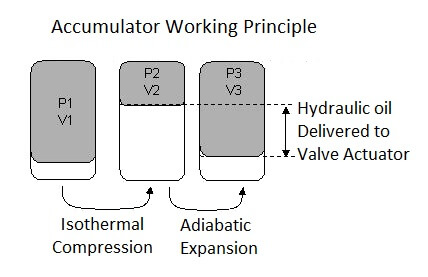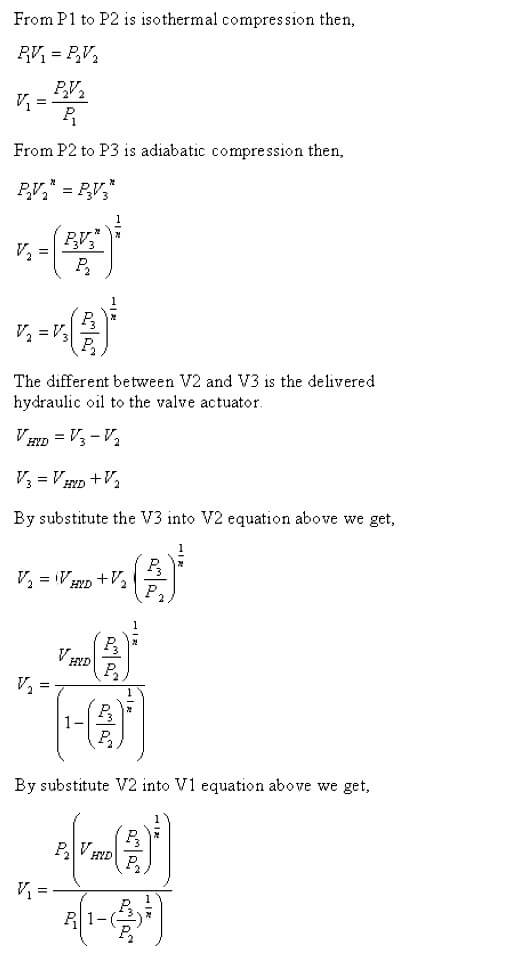Home » Hydraulic Accumulator Sizing for Wellhead Control Panel
Instrumentation Design

# Hydraulic Accumulator Sizing for Wellhead Control Panel

Hydraulic Accumulator Sizing for Wellhead Control Panel Hydraulic Power Unit (Hpu)

In the wellhead control panel there is a Hydraulic Power Unit (HPU) that utilize hydraulic reservoir, hydraulic pumps, and accumulator as it components.

To make the hydraulic demand from SCSSV (Surface Controlled Sub sea Safety Valves) or SSV (Surface Safety Valves) is achievable, the downstream of the hydraulic pump (hydraulic header) is equipped with an accumulator. It helps the hydraulic pump to supply the hydraulic demand quickly (See Introduction to Wellhead Control Panel).

## Hydraulic Accumulator Sizing

Since the accumulator plays a vital role as a hydraulic pressure storage (or energy storage), careful sizing and calculation must be done so that the well operation requirement is achieved. So actually what is the hydraulic accumulator and how to calculate the quantity required?

Hydraulic accumulator is an energy storage device that has gas section and hydraulic oil section. At initial conditions the gas section shall be pre-charged with some gases (usually dry nitrogen) at some pressure.

When the hydraulic oil pumped into the accumulator, the hydraulic oil pressure will press the gas section (gas is compressible while liquid isn’t compressible) into certain volume and certain pressure.

If there is a hydraulic demand, then the accumulator will release the hydraulic oil and makes the gas section expanded. See below drawing for accumulator working principle illustration.Pre-Charge Conditions:

P1 = 0.9 P3 for Bladder accumulators (90% of minimum system pressure to prevent bladder damage)

P1 = P3 for Piston accumulators Charged Conditions:

P3 = Minimum system operating pressure

P2 = Maximum system operating pressure

As per above description, the goal of the sizing is to find the effective volume of the accumulator V1. To avoid any deficiency due to something that we don’t know (un-accurate valve actuator swept volume etc) we need to put some margin (lets say 10% of V1) so the accumulator volume will be 1.1 x V1.

The filling or compression process by the hydraulic pump (from P1 to P2) can be considered as isothermal process since there is no temperature change after compression. While the expansion process (from P2 to P3) can be considered as adiabatic process since the expansion process is fast due to the usage in the valve actuator.

This fast expansion will make the change in heat doesn’t have sufficient time to transferred outside the accumulator. From this assumption, we can develop the following equation to calculate the required effective volume V1 of the accumulator.Where:

P1 = 0.9 P3 for Bladder accumulators (90% of minimum system pressure to prevent bladder damage)
P1 = P3 for Piston accumulators
P2 = Maximum system operating pressure
P3 = Minimum system operating pressure
V1 = Effective gas volume of the accumulator
V2 = Maximum gas volume compressed by the hydraulic oil
V3 = Expanded volume of gas at the minimum pressure required (P2)
VHYD = Amount of oil to be supplied to valve actuator (swept volume of hydraulic valve actuator)
n = ratio of specific heat or CP/CV = 1.4 for nitrogen

### Example :

#### The following data was provided by the x-mast tree vendor:

• 10 well were drilled and supplied complete with SCSSV, Master, and Wing Valve.
• Operating supply pressure to control line of SCSSV actuator is 4500 – 5500 psi
• Operating supply pressure to control line of Master & Wing Valve actuators is 2500 – 3500 psi
• The control line fluid to SCSSV, Master, and Wing Valve actuator is Shell Tellus 32 Hydraulic Oil
• Swept volume of SCSSV is 0.05 ltr, Master & Wing valve is 2.5 ltr.

As per data above, we will have two hydraulic header, one for high pressure (4500 – 5500 psi operating pressure) and one for medium pressure (2500 – 3500 psi operating pressure).

Each header will have its own accumulator to storage the hydraulic pressure so that all valves can be opened twice (this twice opening cycle usually comes from project specification, may be differ on each project) without the present of the hydraulic pump.

The selected accumulator type is bladder type.

P1 = 0.9 * P3 = 4050 psi

P2 = 5500 psi

P3 = 4500 psi

VHYD = 0.05 ltr x 10 SCSSV x 2 opening cycle = 1 Ltr

Then we can get V1 (accumulator gas effective volume) as follow:

V1 = 5500 * (1*(4500/5500)^(1/1.4))/(4050*(1-(4500/5500)^(1/1.4)))
V1 = 8.81 Ltr

By putting 10% margin the V1 will become

V1 = 1.1 x 8.81 Ltr = 9.69 Ltr

By looking at the bladder accumulator vendor catalogue, we can conclude that we need two bladder type accumulators with the following data:

Nominal Capacity = 10 Ltr

Effective Gas Volume = 9.4 Ltr

With this design, we will have almost twice of the effective gas volume surplus. This is good design.

P1 = 0.9 * P3 = 2250 psi

P2 = 3500 psi

P3 = 2500 psi

VHYD = 2.5 ltr x 10 Master Valve x 10 Wing Valve x 2 opening cycle = 100 Ltr

Then we can get V1 (accumulator gas effective volume) as follow:

V1 = 3500 * (100*(2500/3500)^(1/1.4))/(2250*(1-(2500/3500)^(1/1.4)))

V1 = 572.6 Ltr

By putting 10% margin the V1 will become

V1 = 1.1 x 572.6 Ltr = 629.8 Ltr

By looking at the bladder accumulator vendor catalogue, we can conclude that we need 13 bladder type accumulators with the following data:

Nominal Capacity = 54 Ltr

Effective Gas Volume = 49.2 Ltr

With this design, we will have the following surplus of the gas effective volume:

49.2 Ltr x 13 = 9.77 Ltr

#### Articles You May Like :

Instrument Air Compressor

What is Wellhead Control Panel ?

Pressure Transmitter Calibration

Instrumentation Earthing

Resistance Temperature Detectors

#### Basic Principles of Electricity

This website uses cookies to improve your experience. We'll assume you're ok with this, but you can opt-out if you wish. Accept Read More

WordPress Image Lightbox

Send this to a friend